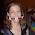## Tuesday, September 05, 2006

### Squares

The number 150 is expressible as the sum of distinct squares, as shown:
150 = 100 + 49 + 1 = 10^2 + 7^2 + 1^2

Every number above 150 is expressible as the sum of distinct squares. But there are 37 numbers that cannot be expressed in this fashion. Care to find the largest one?

1.Ohh... No answer? Did I finally stump you all?

128 is the largest number: Note that 128-100 = 28 which cannot be expressed. But 29 - 50 can be (for instance 29 = 25 + 4, 30 = 25 + 4 + 1, etc...)

2.I was thinking of a mathematical way to solve this, since every square can be expressed as a sum of consecutive odd numbers:

1^2=1
2^2=4=1+3
3^2=9=1+3+5
4^2=16=1+3+5+7
etc.

since (n+1)^2=n^2+2n+1, meaning that (n+1)^2 is 2n+1 greater than n^2, which is where the consecutive odd numbers come from. Therefore, every square can be expressed like the sum from n=0 to some number k of (2n+1). Anyway, there has got to be some way to prove this like that, but I didn't have time yesterday. Have you tried that, Mike? Any thoughts?

3.Abe, I'll have to think about that some more.

4.Since you told us there are 37 duds, lets find them. The 37th will be it.

2, 6, 7, 8, 11, 12, 15, 18, 19, 22
(that's ten)
23, 24, 27, 28, 31, 32, 33, 40, 43,

[Digression: is 44 on the list? 44 = 36 + 8, but 8 is on the list. 44 = 25 + 19, but 19 is on the list. 44 = 16 + 28....]

44
(that's twenty)
47, 48, 51, 55, 60, 67, 72, 76, 88, 92,

(that's thirty)
96, 108, 112, 115, 124, 128.

Where'd I goof? I only got 36 up to 128.

5.Jonathan you forgot 3

Leave your answer or, if you want to post a question of your own, send me an e-mail. Look in the about section to find my e-mail address. If it's new, I'll post it soon.

Please don't leave spam or 'Awesome blog, come visit mine' messages. I'll delete them soon after.

Enter your Email and join hundreds of others who get their Question of the Day sent right to their mailbox

The Lamplight Manor Puzz 3-D